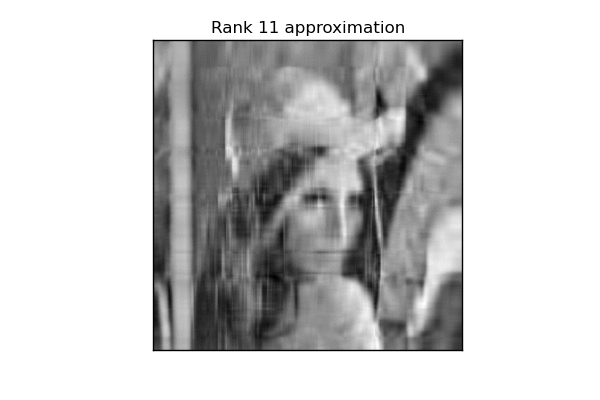# fa.bianp.net

My latest contribution for scikit-learn is an implementation of the isotonic regression model that I coded with Nelle Varoquaux and Alexandre Gramfort …

Besides performing a line-by-line analysis of memory consumption, memory_profiler exposes some functions that allow to retrieve the memory consumption of a function in real-time, allowing e.g. to visualize the memory consumption of a given function over time.

The function to be used is memory_usage. The first argument specifies what …

SciPy contains two methods to compute the singular value decomposition (SVD) of a matrix: scipy.linalg.svd and scipy.sparse.linalg.svds. In this post I'll compare both methods for the task of computing the full SVD of a large dense matrix.

The first method, scipy.linalg.svd, is perhaps …

This tutorial introduces the concept of pairwise preference used in most ranking problems. I'll use scikit-learn and for learning and matplotlib for …

My newest project is a Python library for monitoring memory consumption of arbitrary process, and one of its most useful features is the line-by-line analysis of memory usage for Python code. I wrote a basic prototype six months ago after being surprised by the lack of related tools. I wanted …

A little experiment to see what low rank approximation looks like. These are the best rank-k approximations (in the Frobenius norm) to the a natural image for increasing values of k and an original image of rank 512.Python code can be found here. GIF animation made using ImageMagic's convert …

In scipy's development version there's a new function closely related to the QR-decomposition of a matrix and to the least-squares solution of a linear system. What this function does is to compute the QR-decomposition of a matrix and then multiply the resulting orthogonal factor by another arbitrary matrix. In pseudocode …

As a warm-up for the upcoming EuroScipy-conference, some of the scikit-learn developers decided to gather and work together for a couple of days. Today was the first day and there was only a handfull of us, as the real kickoff is expected tomorrow. Some interesting coding happened, although most of …

I was last weekend in FOSDEM presenting scikits.learn (here are the slides I used at the Data Analytics Devroom). Kudos to Olivier Grisel and all the people who organized such a fun and authentic meeting!